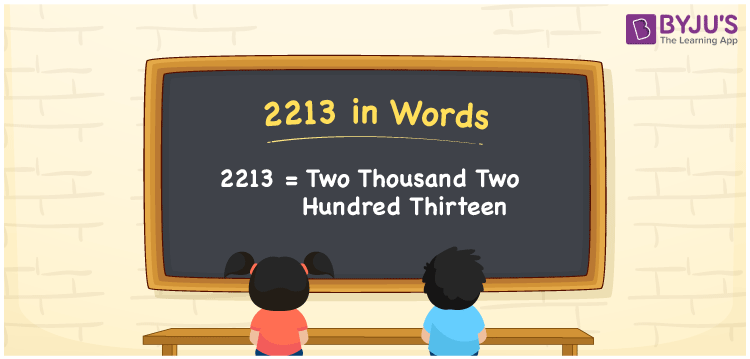# 2213 in Words

2213 in words is written as Two thousand two hundred thirteen. In both the International System of Numerals and the Indian System of Numerals, 2213 is written as Two thousand two hundred thirteen. The number 2213 is a Cardinal Number as it could represent some quantity. For example, “this video got 2213 likes”.

 2213 in Words Two thousand two hundred thirteen Two thousand two hundred thirteen in Number 2213

## 2213 in English Words

2213 in English words is read as “Two thousand two hundred thirteen”.## How to Write 2213 in Words?

To write 2213 in words, we shall use the place value chart. In the place value chart, put 2 in the thousands, 2 in the hundreds, 1 in the tens, and 3 in the ones, respectively. Let us make a place value chart to write the number 2213 in words.

 Thousands Hundreds Tens fours 2 2 1 3

Thus, we can write the expanded form as

2 × Thousand + 2 × Hundred + 1 × Ten + 3 × One

= 2 × 1000 + 2 × 100 + 1 × 10 + 3 × 1

= 2000 + 200 + 10 + 3

= 2213

= Two thousand two hundred thirteen.

2213 is a natural number, the successor of 2212 and the predecessor of 2214.

2213 in words – Two thousand two hundred thirteen

• Is 2213 an odd number? – Yes
• Is 2213 an even number? – No
• Is 2213 a perfect square number? – No
• Is 2213 a perfect cube number? – No
• Is 2213 a prime number? – Yes
• Is 2213 a composite number? – No

## Frequently Asked Questions on 2213 in Words

Q1

### How to write 2213 in words?

2213 in words is written as Two thousand two hundred thirteen.
Q2

### How to write 2213 in the International and Indian System of Numerals?

In both, the system of numerals, 2213 in words, is written as Two thousand two hundred thirteen.
Q3

### How to write 2213 in a place value chart?

In the place value chart, write 2 in the thousands, 2 in the hundreds, 1 in the tens, and 3 in the ones, respectively.